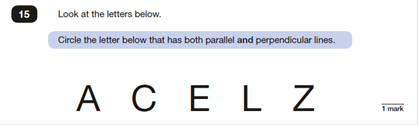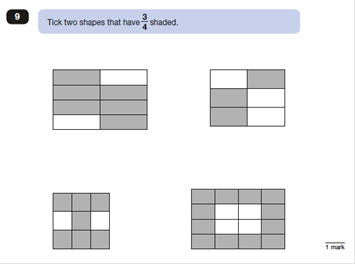# SATs Reasoning Blog 1: Tackling Selected Response QuestionsThe 2018 KS2 SATs are fast approaching and, like last year, pupils will be required to take three mathematics papers. The three papers will focus on two key components: (1) arithmetic and (2) reasoning.

I am sure most of you would agree that preparation for the first paper, arithmetic, is far easier than preparing for papers 2 and 3. The reason being is that what will be assessed and the format of the questions in paper 1 is quite predictable. On the other hand, the reasoning papers prove more of a challenge as mathematical problems are presented in a wide range of formats. This is to ensure pupils can fully demonstrate mathematical fluency, mathematical problem solving and mathematical reasoning.

With 70 marks out of a total of 110 marks being awarded to pupils' knowledge and understanding of mathematical reasoning, it is critical that pupils have regular opportunities to practise the range of question types that can potentially arise.  What we do know is that the reasoning questions will be presented as either selected response questions or constructed response questions.

Selected response questions require pupils to select which option satisfies the constraint given in the question, whereas constructed response questions require pupils to construct an answer rather than simply select one or more options.

The range of questions include:

 Selected Response Constructed Response multiple choice matching true/false or yes/no completing a chart or table drawing a shape show your method evaluate a statement

Preparation for the reasoning tests should involve pupils being exposed to practising a range of these question types. Often, however, this is not approached systematically and pupils are not explicitly shown how to answer the range of question types that can appear.

In 2017, there were 47 reasoning questions across papers 2 and 3 of which 8 questions were selected response. The remaining questions (i.e. 39 questions) all involved pupils constructing their response.

The new Problem Solving and Reasoning series focuses on teaching strategies to answer the following range of questions that either involve selecting or constructing a response:

1. Matching

2. Ordering

3. Multiple choice

4. True or false

5. Missing number

6. Explain how you know

7. Real-life multi-step word problems

1. Completing tables and charts

2. Data interpretation

In this first blog, out of a series of two, we will consider selected response questions. It may be considered that selected response questions such as multiple choice questions will be successfully answered by the majority of pupils. However, the question below from the 2017 SATS was answered correctly by only 56% of pupils.Of course, some pupils would have answered this incorrectly because they did not know the meaning of parallel and perpendicular lines.  But there were some pupils who incorrectly circled more than one letter and did not indicate the correct answer clearly enough.  This could be prevented if pupils were taught the technique of answering this question.

Another multiple choice selected response question that appeared in the 2017 tests was Q9 Paper 2, which involved pupils ticking two out of the four shapes.It is therefore important to clearly model to pupils how to decipher what the question is asking for and demonstrate how to accurately answer the question. Interestingly, last year, 7 out of 8 selected response questions involved multiple choice.

It is worth remembering that selected response questions can also be more open-ended in nature. For example, Paper 3 Q3 required pupils to select the digit cards to make a 2-digit x 1-digit number sentence that gives an answer that is a multiple of 10.

In preparation for 2018, consider if your pupils are being given an opportunity to practise the following selected response question types:

• Matching
• True/false or Yes/No
• Multiple choice  - circling, crossing or ticking the correct answer or answers
• Selecting the correct digits to complete number sentences or facts

It is important to remember that it is key to explicitly demonstrate and model to pupils how they should answer such questions. This will put them in good stead for the forthcoming SATs tests!

Sarah-Anne Fernandes is a primary mathematics consultant and author of our Reasoning Practice Tests series.

Loved this blog? Get this content straight to your inbox and sign-up to our e-updates. Simply create an account using this link and select Maths as your subject preference.

### Tags

mathematics, maths, reasoning

x